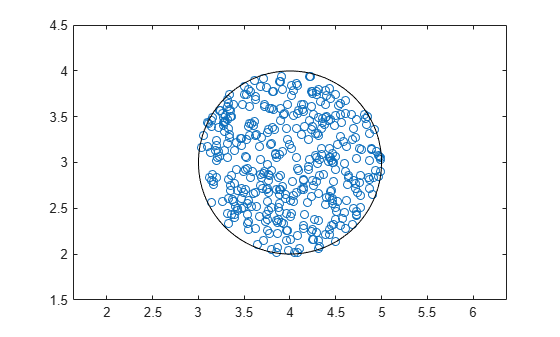ucomplex

Create uncertain complex parameter

Syntax

A = ucomplex('NAME',nominalvalue)
A = ucomplex('NAME',nominalvalue,'Property1',Value1,...
'Property2',Value2,...)

Description

An uncertain complex parameter is used to represent a complex number whose value is uncertain. Uncertain complex parameters have a name (the Name property), and a nominal value (the NominalValue property).

The uncertainty (potential deviation from the nominal value) is described in two different manners:

• Percentage (disc size is percentage of magnitude of NominalValue)

The Mode property determines which description remains invariant if the NominalValue is changed (the other is derived). The default Mode is 'Radius' and the default radius is 1.

Property/Value pairs can also be specified at creation. For instance,

B = ucomplex('B',6-j,'Percentage',25)

sets the nominal value to 6-j, the percentage uncertainty to 25 and, implicitly, the Mode to 'Percentage'.

Examples

collapse all

Compute 400 random samples of an uncertain complex parameter and visualize them in a plot.

Create an uncertain complex parameter with internal name A.

A = ucomplex('A',4+3*j)
A =
Uncertain complex parameter "A" with nominal value 4+3i and radius 1.

The uncertain parameter's possible values are a complex disc of radius 1, centered at 4 + 3_j_. The value of A.percentage is 20 (radius is 1/5 of the magnitude of the nominal value).

You can visualize the uncertain complex parameter by sampling and plotting the data.

sa = usample(A,400);
w = linspace(0,2*pi,200);
circ = sin(w) + j*cos(w);
rc = real(A.NominalValue+circ);
ic = imag(A.NominalValue+circ);
plot(real(sa(:)),imag(sa(:)),'o',rc,ic,'k-')
xlim([2.5 5.5])
ylim([1.5 4.5])
axis equal